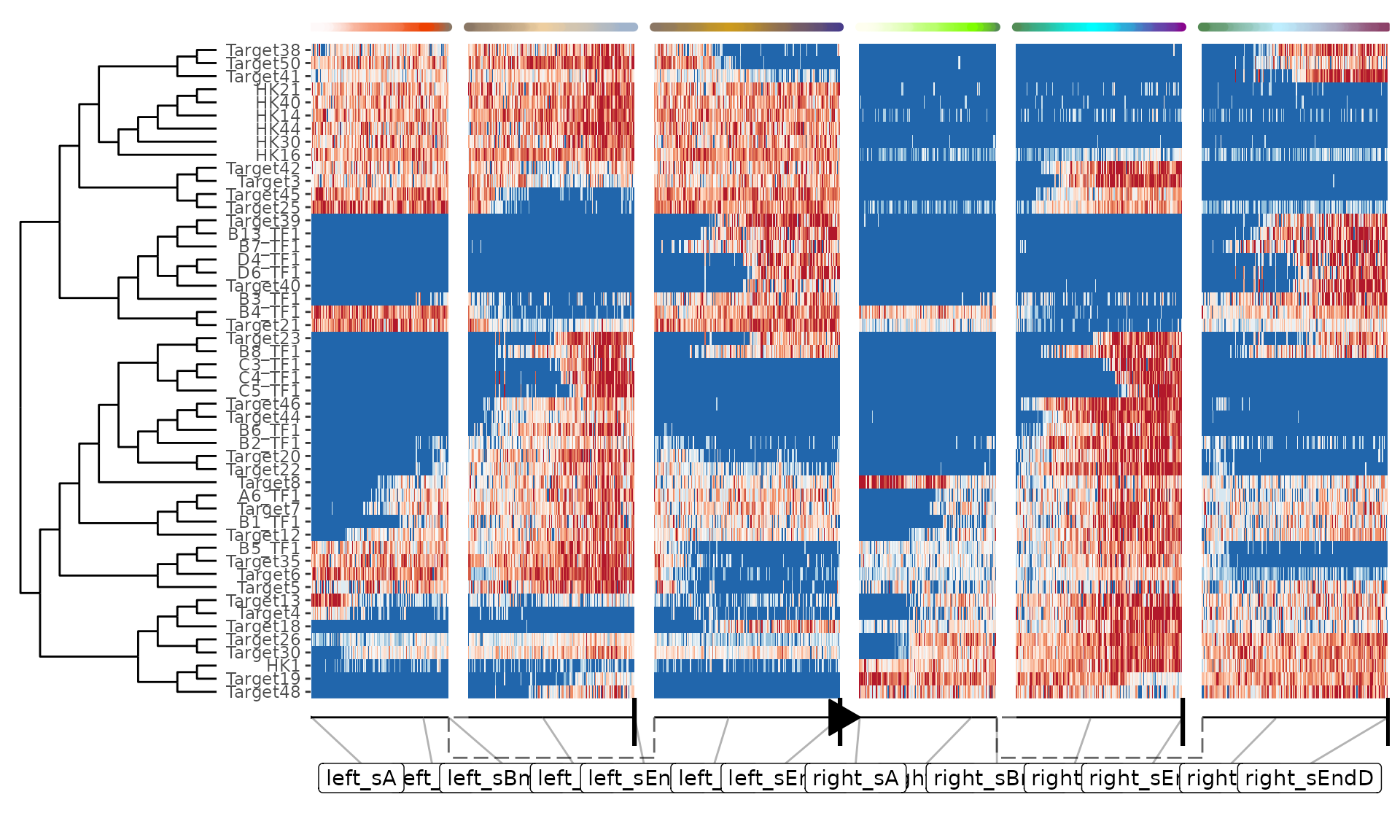An easy way of simulating batch effects is by performing multiple dyngen runs, but with different kinetics. dyngen samples the GRN kinetics randomly from a set of predefined distributions (See ?generate_kinetics).

## Create underlying gene regulatory network

First, create the ‘common’ part of the dyngen simulation as follows. This will ensure that the gene regulatory network-part of the simulation is exactly the same.

library(tidyverse)
library(dyngen)

set.seed(1)

backbone <- backbone_bifurcating()
config <-
initialise_model(
backbone = backbone,
num_cells = 1000,
num_tfs = nrow(backbone$module_info), num_targets = 250, num_hks = 250, simulation_params = simulation_default( census_interval = 10, ssa_algorithm = ssa_etl(tau = 300 / 3600), experiment_params = simulation_type_wild_type(num_simulations = 100) ) ) # the simulation is being sped up because rendering all vignettes with one core # for pkgdown can otherwise take a very long time set.seed(1) config <- initialise_model( backbone = backbone, num_cells = 1000, num_tfs = nrow(backbone$module_info),
num_targets = 50,
num_hks = 50,
verbose = interactive(),
simulation_params = simulation_default(
census_interval = 5,
ssa_algorithm = ssa_etl(tau = .01),
experiment_params = simulation_type_wild_type(num_simulations = 10)
)
)
model_common <-
config %>%
generate_tf_network() %>%
generate_feature_network()

## Simulate multiple times with different kinetics

Now you can run the simulation multiple times. Note that for each separate run, the generate_kinetics() step ensures that the thermodynamic parameters will be different (yet drawn from the same distribution). Some values for the kinetics of the main transcription factors are not changed, to ensure that the desired dynamic process is obtained.

model_a <- model_common %>%
generate_kinetics() %>%
generate_gold_standard() %>%
generate_cells()
model_b <- model_common %>%
generate_kinetics() %>%
generate_gold_standard() %>%
generate_cells()

The differences if kinetics parameters can be visualised as follows.

params_a <-
dyngen:::.kinetics_extract_parameters_as_df(model_a$feature_info, model_a$feature_network) %>%
select(id, group = param, model_a = value)

params_b <-
dyngen:::.kinetics_extract_parameters_as_df(model_b$feature_info, model_b$feature_network) %>%
select(id, group = param, model_b = value)

params_ab <-
inner_join(params_a, params_b, by = c("id", "group"))

ggplot(params_ab) +
geom_point(aes(model_a, model_b, colour = group)) +
facet_wrap(~group, scales = "free") +
theme_bw()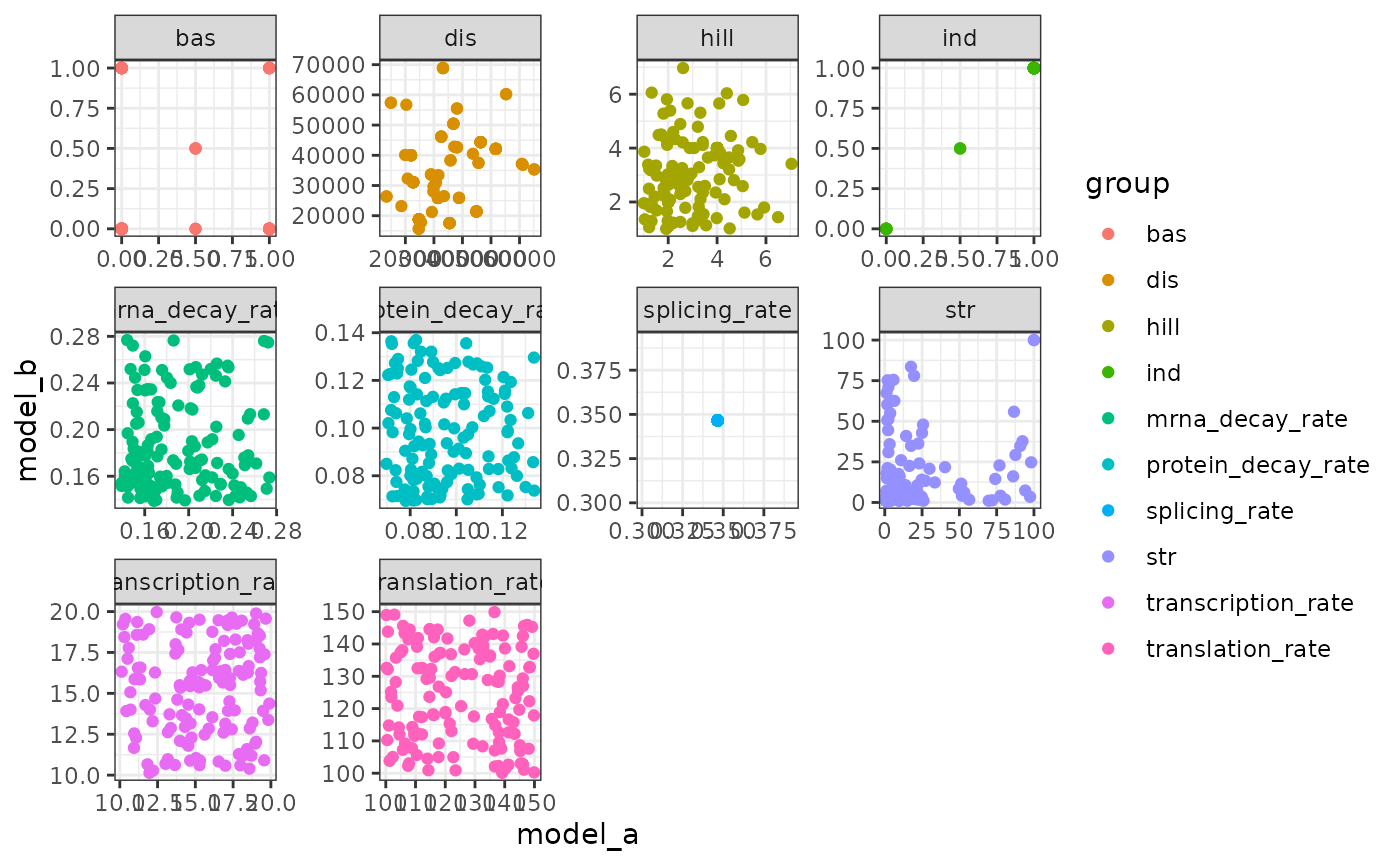## Combine outputs and visualise

Finally, combine the simulations as follows.

model_ab <-
combine_models(list(left = model_a, right = model_b)) %>%
generate_experiment()
## Warning in params$fun(network = network, sim_meta = sim_meta, params = model$experiment_params, : One of the branches did not obtain enough simulation steps.
##   Increase the number of simulations (see ?simulation_default) or decreasing the census interval (see ?simulation_default).

Show a dimensionality reduction.

plot_simulations(model_ab)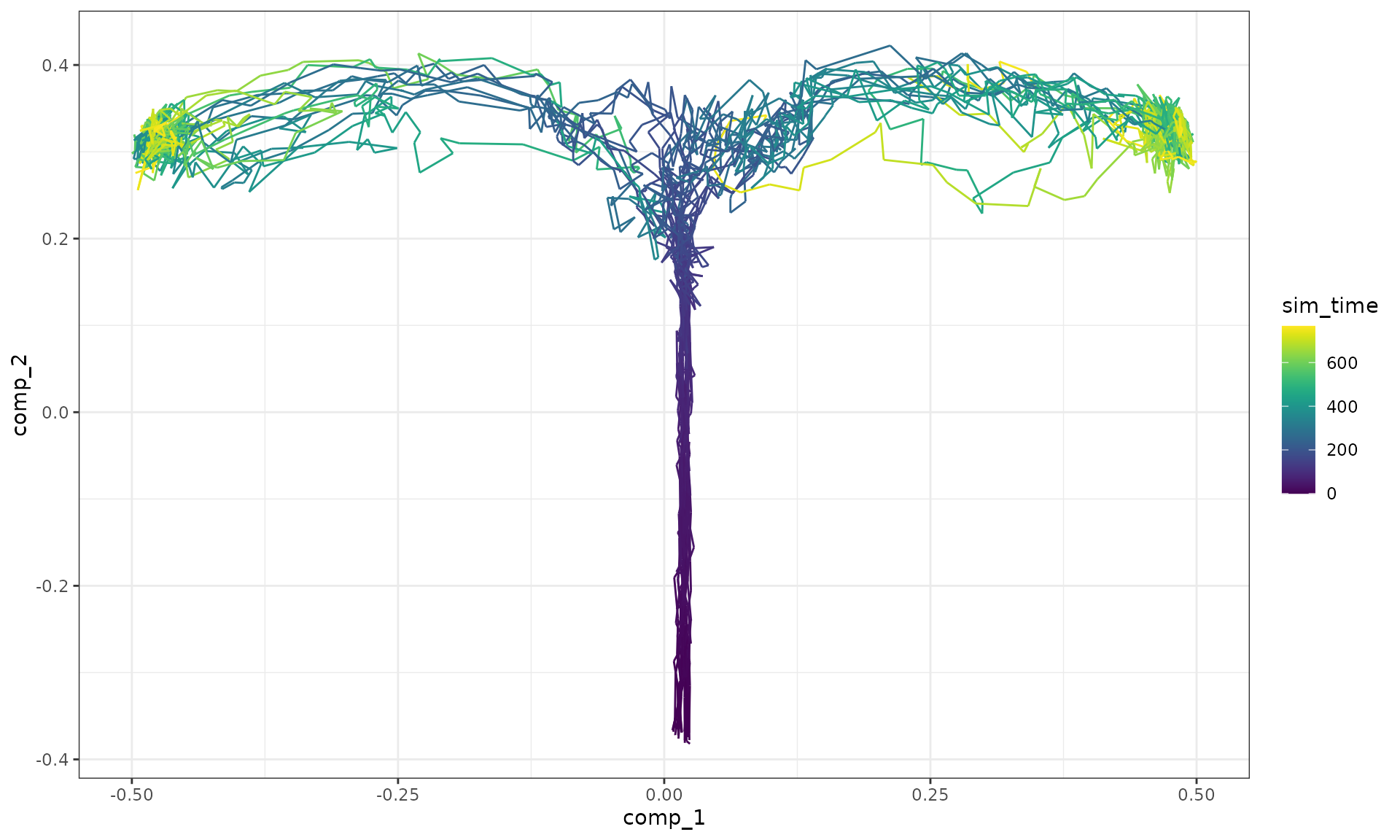plot_gold_mappings(model_ab, do_facet = FALSE)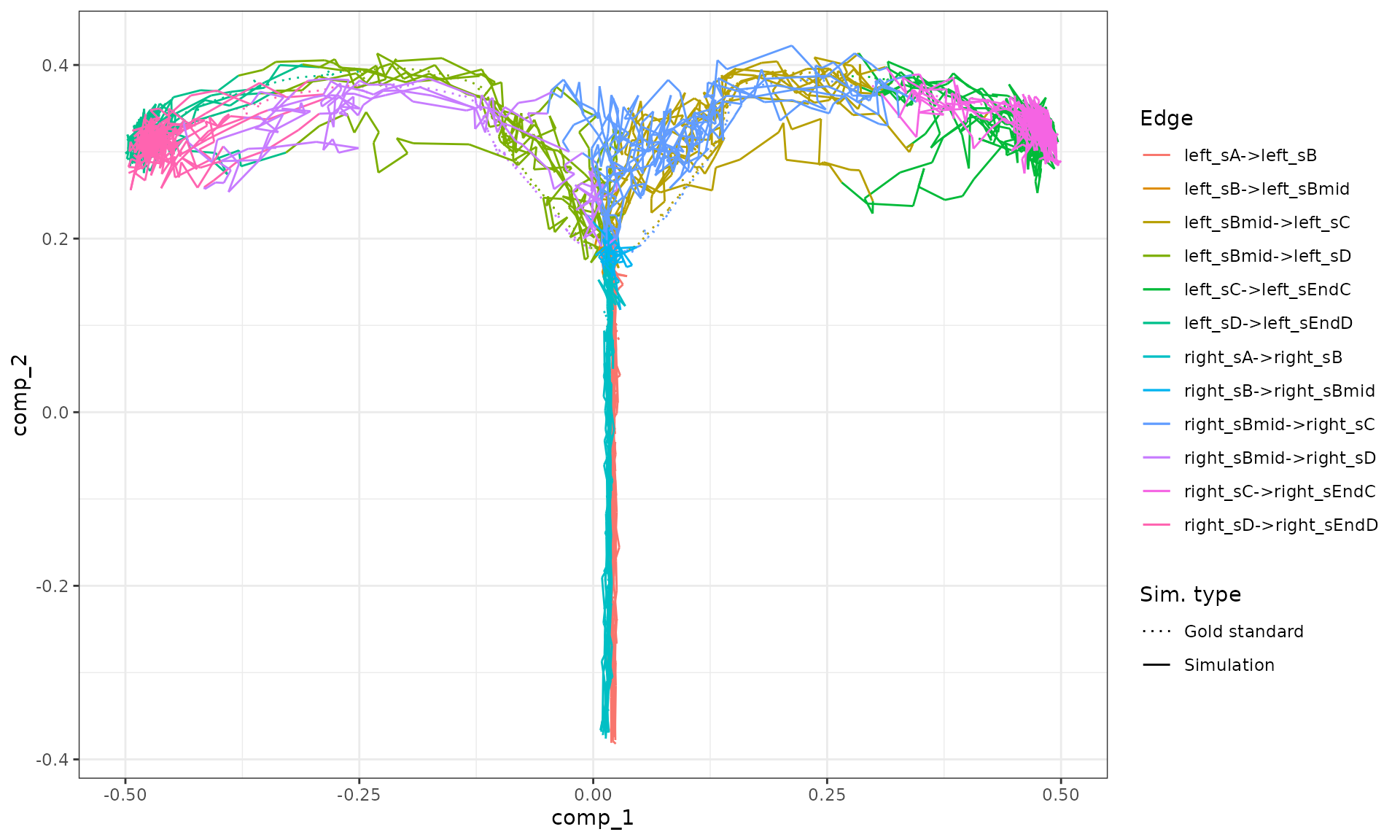Visualise the dataset using dyno.

library(dyno)
## Loading required package: dynfeature
## Loading required package: dynguidelines
## Loading required package: dynmethods
## Loading required package: dynplot
## Loading required package: dynwrap
dataset <- as_dyno(model_ab)
plot_dimred(dataset)
## Coloring by milestone
## Using milestone_percentages from trajectory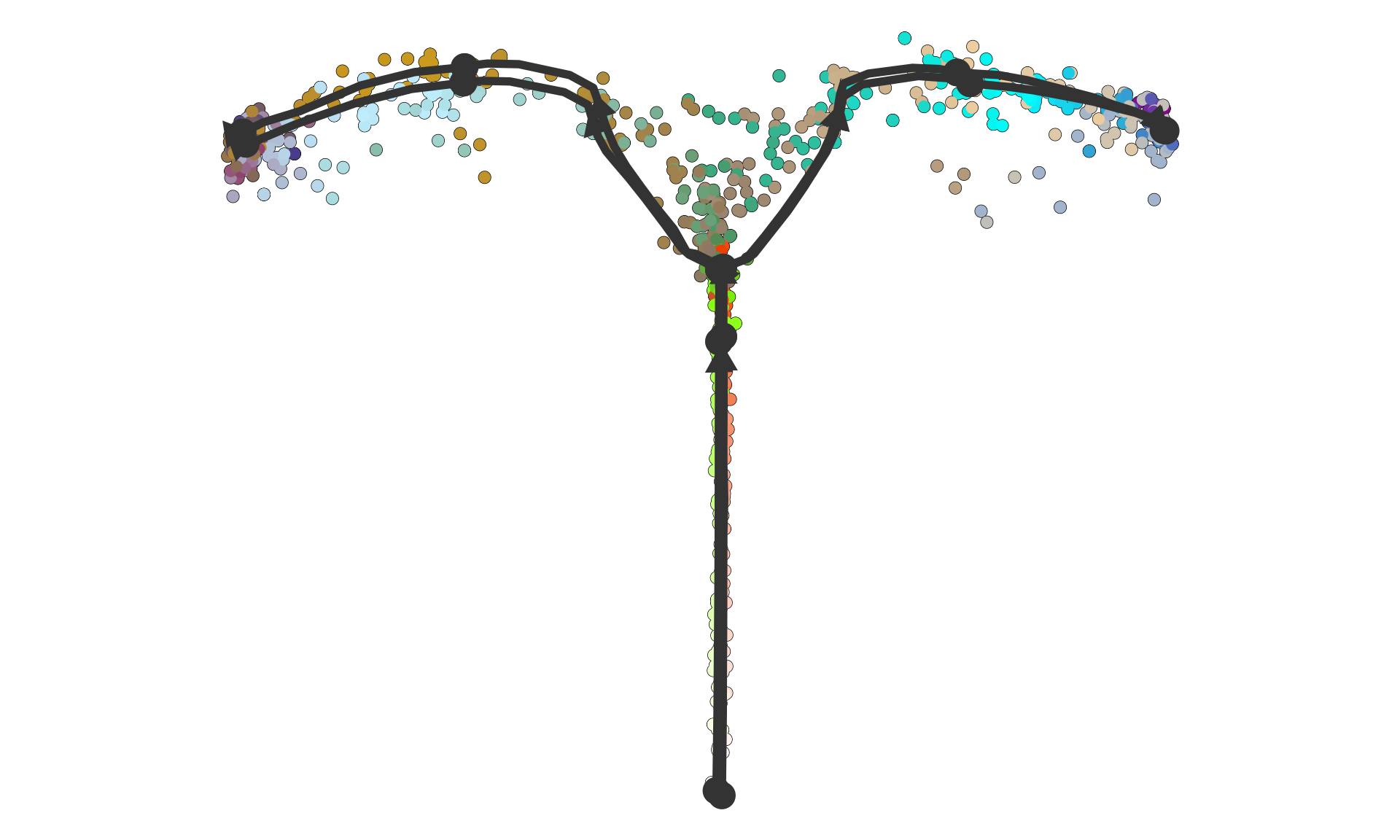plot_heatmap(dataset, features_oi = 50)
## No features of interest provided, selecting the top 50 features automatically
## Using dynfeature for selecting the top 50 features
## root cell or milestone not provided, trying first outgoing milestone_id
## Using 'left_sA' as root
## Coloring by milestone